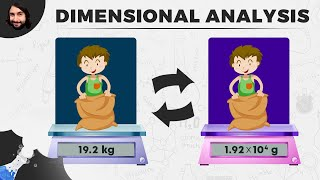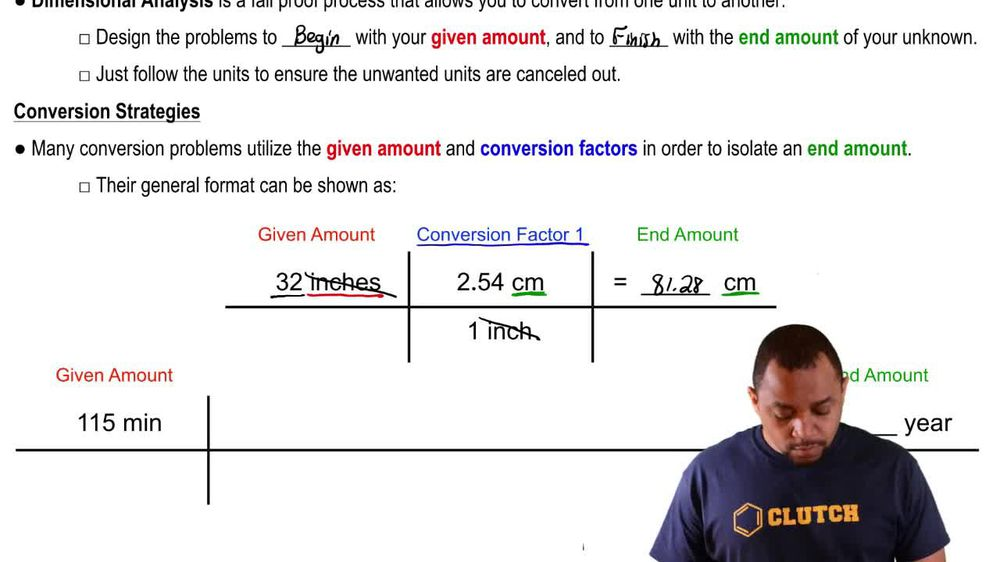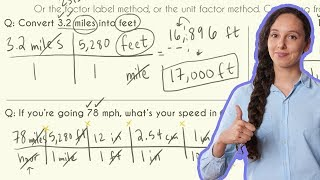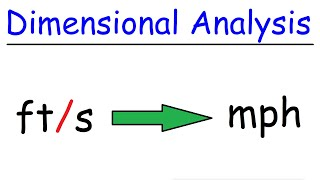Start typing, then use the up and down arrows to select an option from the list.
1. 1. Intro to General Chemistry2. Dimensional Analysis
Problem

# Convert the following quantities into SI units with the correct number of significant figures. (a) 5.4 in.

Relevant Solution1m
Play a video:
Hey everyone. So here it says the height of a mountain is 2,786 ft. What is its height? In S. I. Units? Well, feet is a unit of length and the S. I. Unit for length is meters. So really it's asking us to convert our feet into meters by default. This would mean that option A is our correct answer because it's the only one with meters as the units. But let's work it out and see how it's done. We have here 20,786 ft. We'd say here that one ft Is equal to 12". And then we can say that 1" is equal to 2.54 cm. And if we know centimeters, we can just use metric prefix conversion knowledge to convert it into meters and that is one centimeter is 10 to the negative two m. So that comes out to 6335 . m as our final answer. So this shows how A is the correct answer. Remember when it comes to link the S. I. Unit is in meters. So again, by default option A would be the correct answer.